Dedicated to those who never had the opportunity to receive a good education
Dedicated to those who never had the opportunity to receive a good education
0%

# The Simple Harmonic Oscillator

The one-dimensional simple harmonic oscillator is described by the differential equation

(1)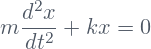Here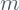is the mass of the particle and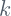is the constant characterizing the restoring force.

## Solution of the simple harmonic oscillator

The solution satisfying the initial condition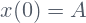, where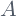is the amplitude of the oscillations, is given by

(2)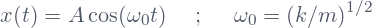The oscillator is called harmonic because it is characterized by a unique frequency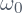and the superior harmonics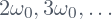do not appear in the solution.

## Total energy of the simple harmonic oscillator

Multiplying Eq.(1) by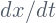and taking into account the relations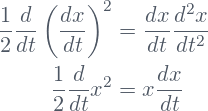Eq.(1) can be rewritten in the form

(3)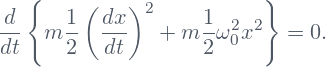The first integral can be easily calculated:

(4)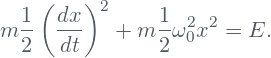In the latter equation the constant of integration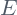is the total energy of the oscillator. The total energy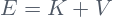is the sum of the kinetic energy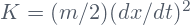and the potential energy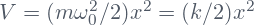.

Substituting in Eq.(4) the solution given by Eq.(2) one finds that the total energyis characterized by the square of the amplitudeand does not depend on the frequency of the oscillations:

(5)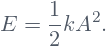## Comparison with the quantum harmonic oscillator

The amplitude, and consequently the energycan take any value. This is in contrast with the quantum harmonic oscillator in which the energy can take only discrete values, doesn’t depend on the amplitude, and is proportional to the frequency of the oscillations (see QHO).

Substituting Eq.(2) in the expression for the momentum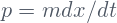one obtains

(6)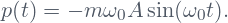It is clear from the equations (2) and (6) that the position and the momentum of the classical simple harmonic oscillator can take well defined values simultaneously at each instant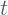. This is also in contrast with the quantum harmonic oscillator in which these couple of dynamical variables satisfy the uncertainty relation at each instant(see QHO).

## Hamiltonian of the simple harmonic oscillator

The Hamiltonian for the harmonic oscillator can be written in the form

(7)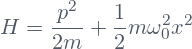Share it!